# Digit Cards 1 10 PrintableUpdated on Jun 23, 2022By Printablee Team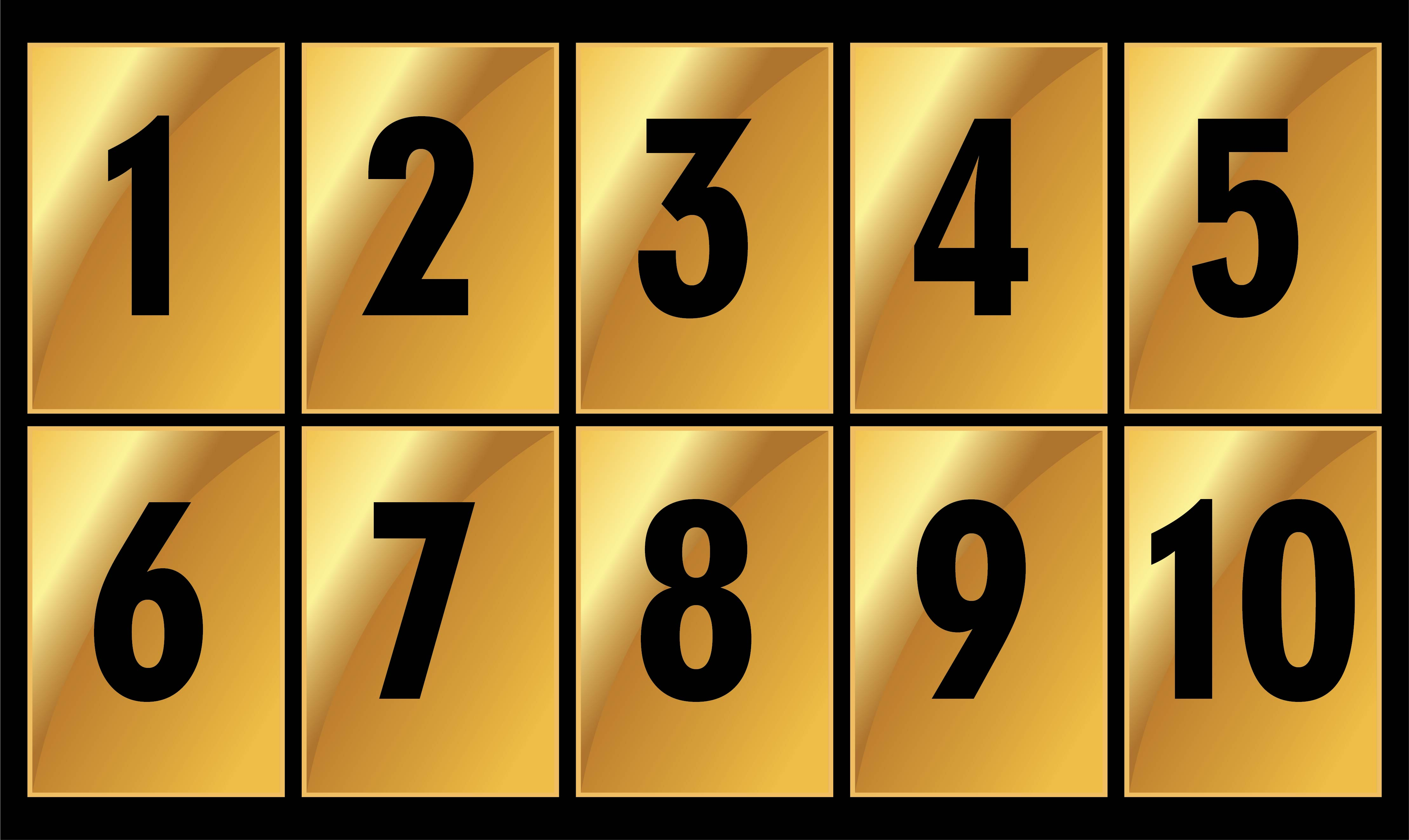### What are Digit cards?

Digit cards refer to a set of cards that contains numbers. Each card only contains a certain number. The form of the digitals is actually similar to number flash cards. To introduce numbers to kids with the beginner level of numbers, we can use digit cards 1-10. It's because they only need to learn with fewer numbers in the beginning.

Too many lessons will make them overwhelmed. Digit cards are suitable for younger children to middle schoolers. The digit cards also help kids to understand and or differ the concept of the digits and numbers.

### What is an example of a digit?

A digit can be defined as a symbol to represent numbers or to portray numerical information. When we see the various numbers, starting from 0, 1, 2, 3, 4, 6, 7, 8, and 9, those can be determined as a digit. If we see a single symbol between 1 to 9, then the numeral can be called a 1 digit.

If we want to name something in hundreds, we will be able to see that there are three kinds of symbols. Then, it can be defined as three digits of numbers. The number of digits is calculated based on the number of numeric symbols that appear. We can download digit cards 1-10 to get our own card as a device for introducing kids to the concept of digits.

### What does 3 digit number mean?

Three-digit numbers consist of hundreds, tens, and ones. The numbers representing the hundreds are written on the far left. Followed by the tens in the middle. Finally, on the far right are the numbers for the ones. The greater the value of a number, the more it is positioned to the left. Let's look at an example of a three-digit number: 345.

At first glance, the numbers are in order from smallest to largest value. In fact, it is the number 3 that has the greatest value, namely the value of hundreds of three hundred. Then, the smaller value in the three-digit number is denoted by the number 4, which is forty. In this case, the number 5 actually represents the lowest value, namely the unit. In simple form, the three-digit number 345 is made up of three hundred plus forty and plus five.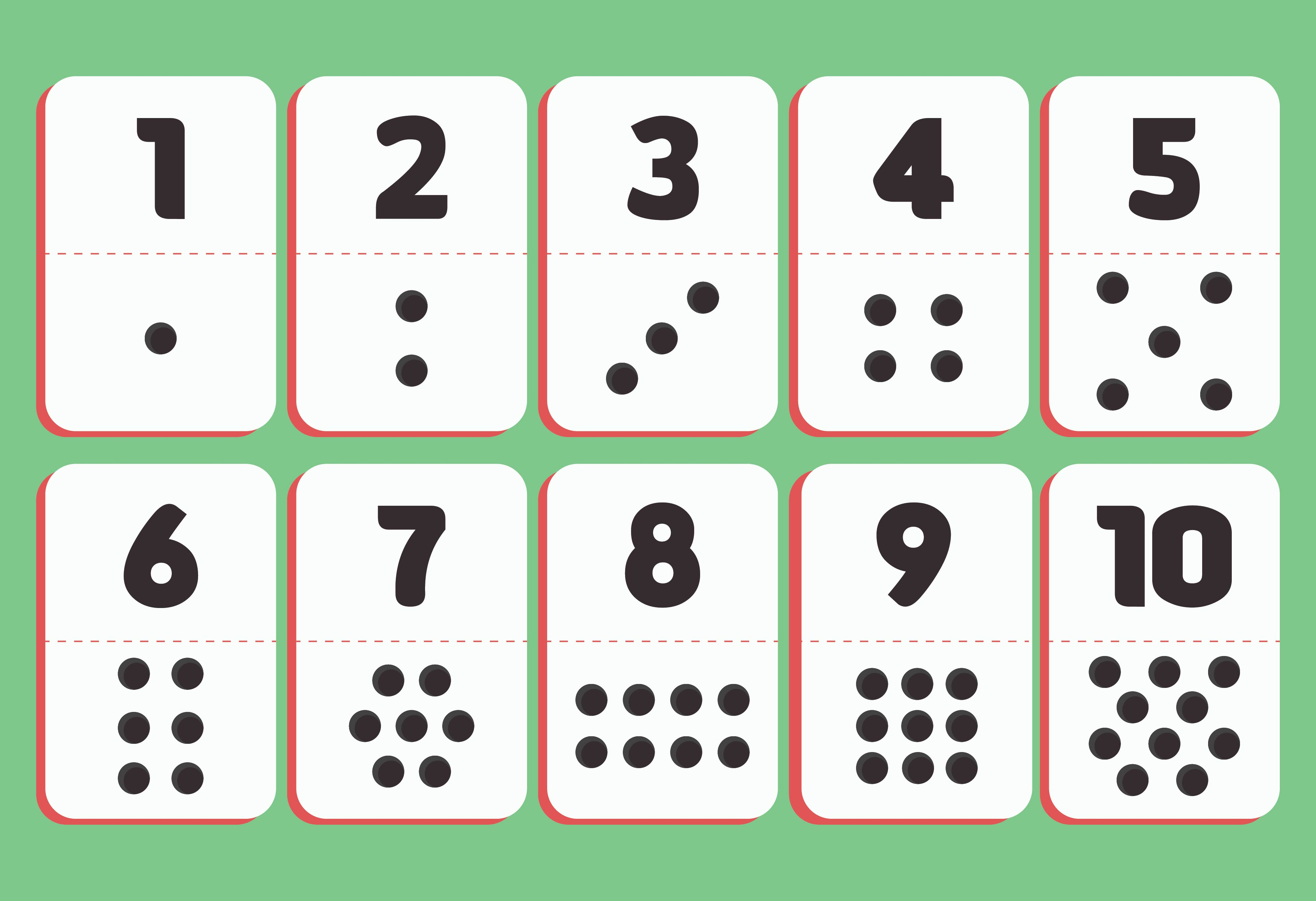We also have more printable card you may like:
Printable Number Cards
Classic Bingo Cards Printable
Pokemon Card Checklist Printable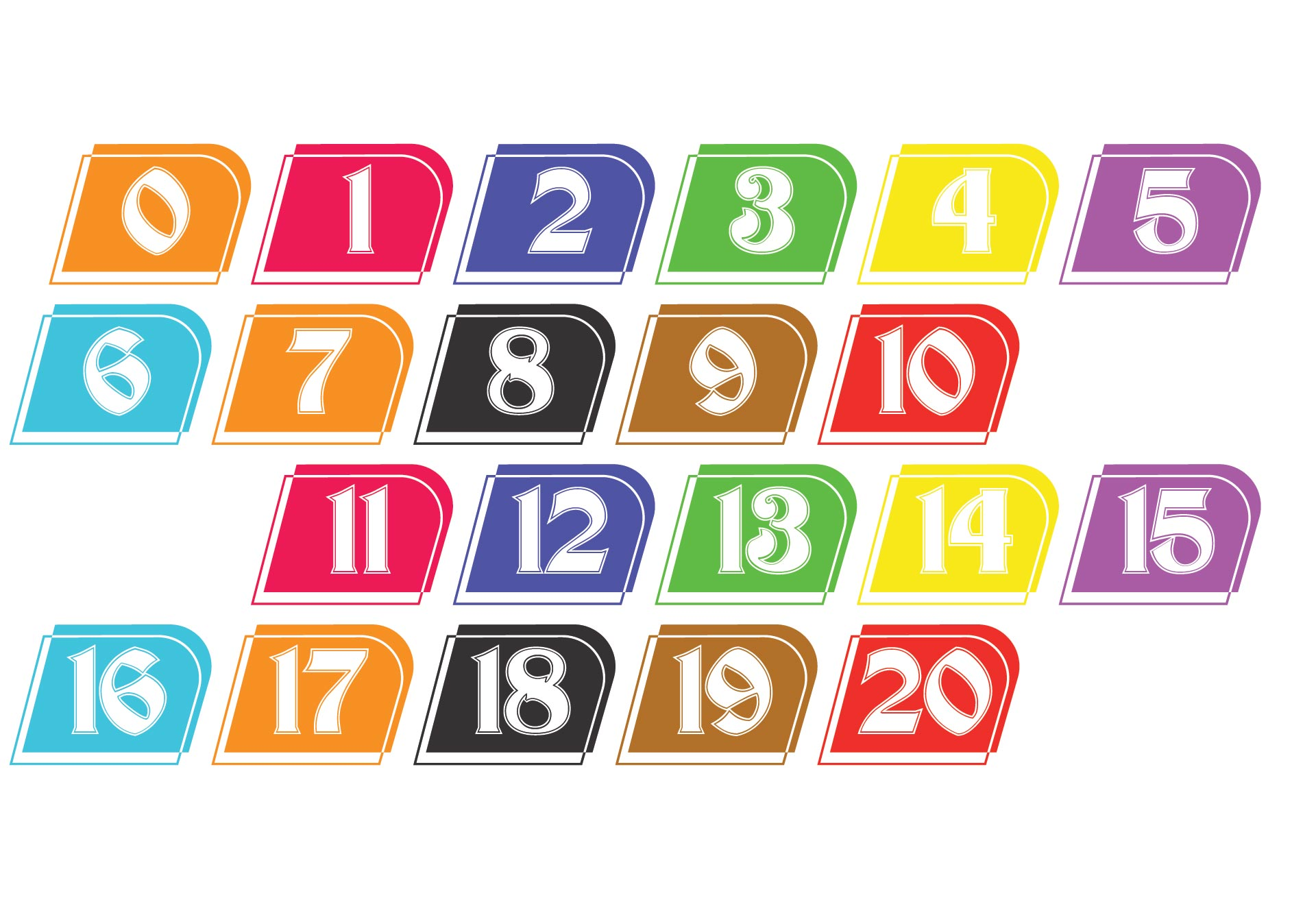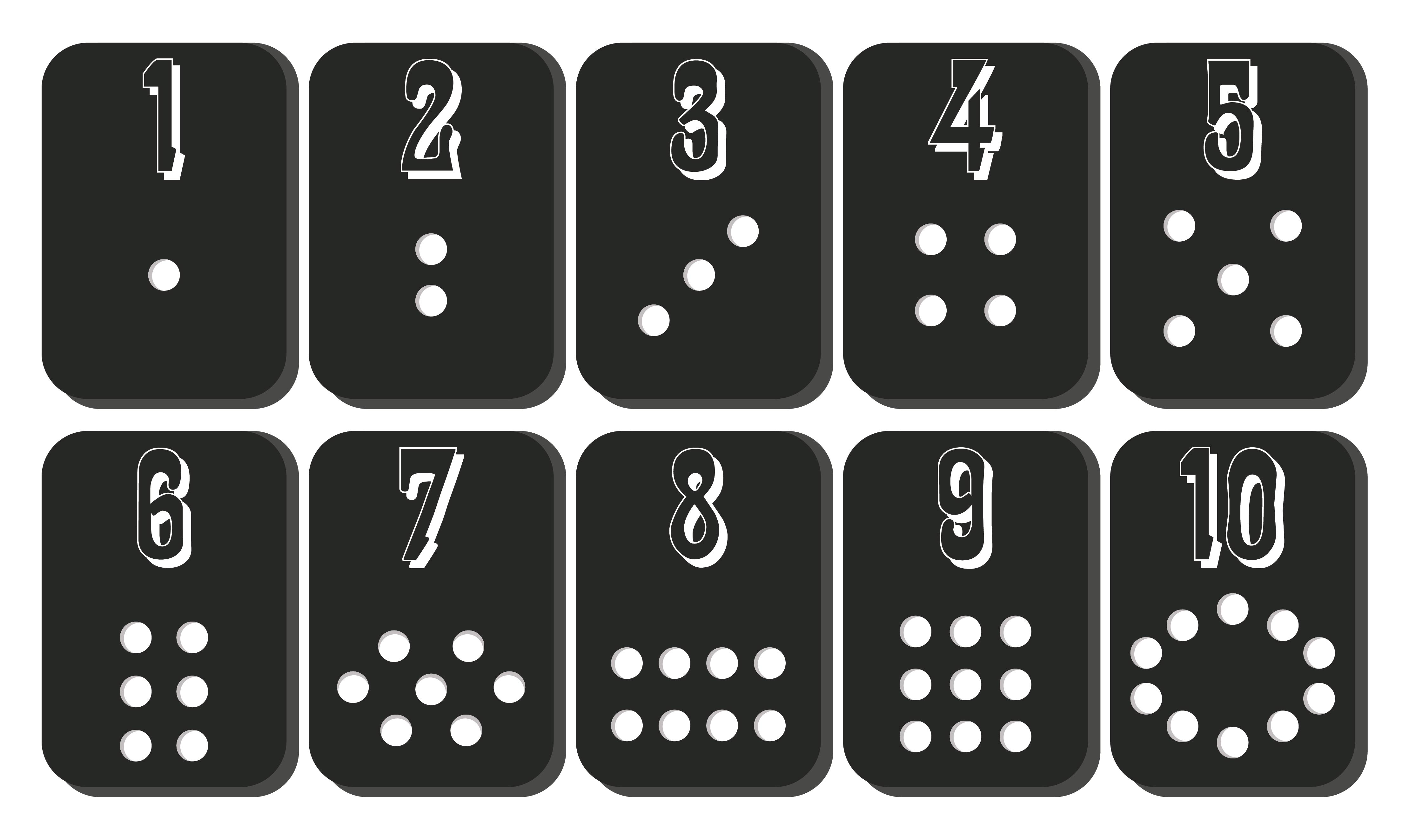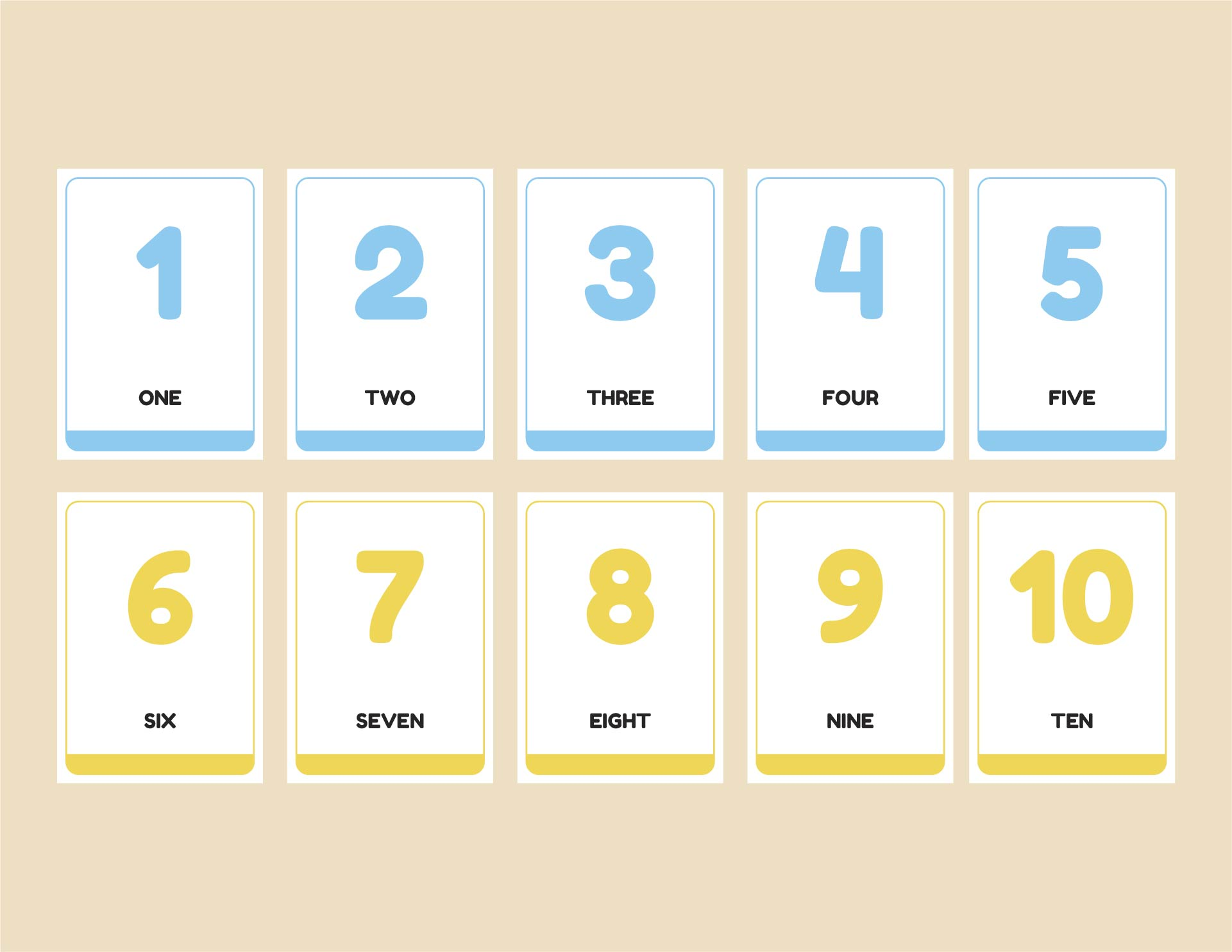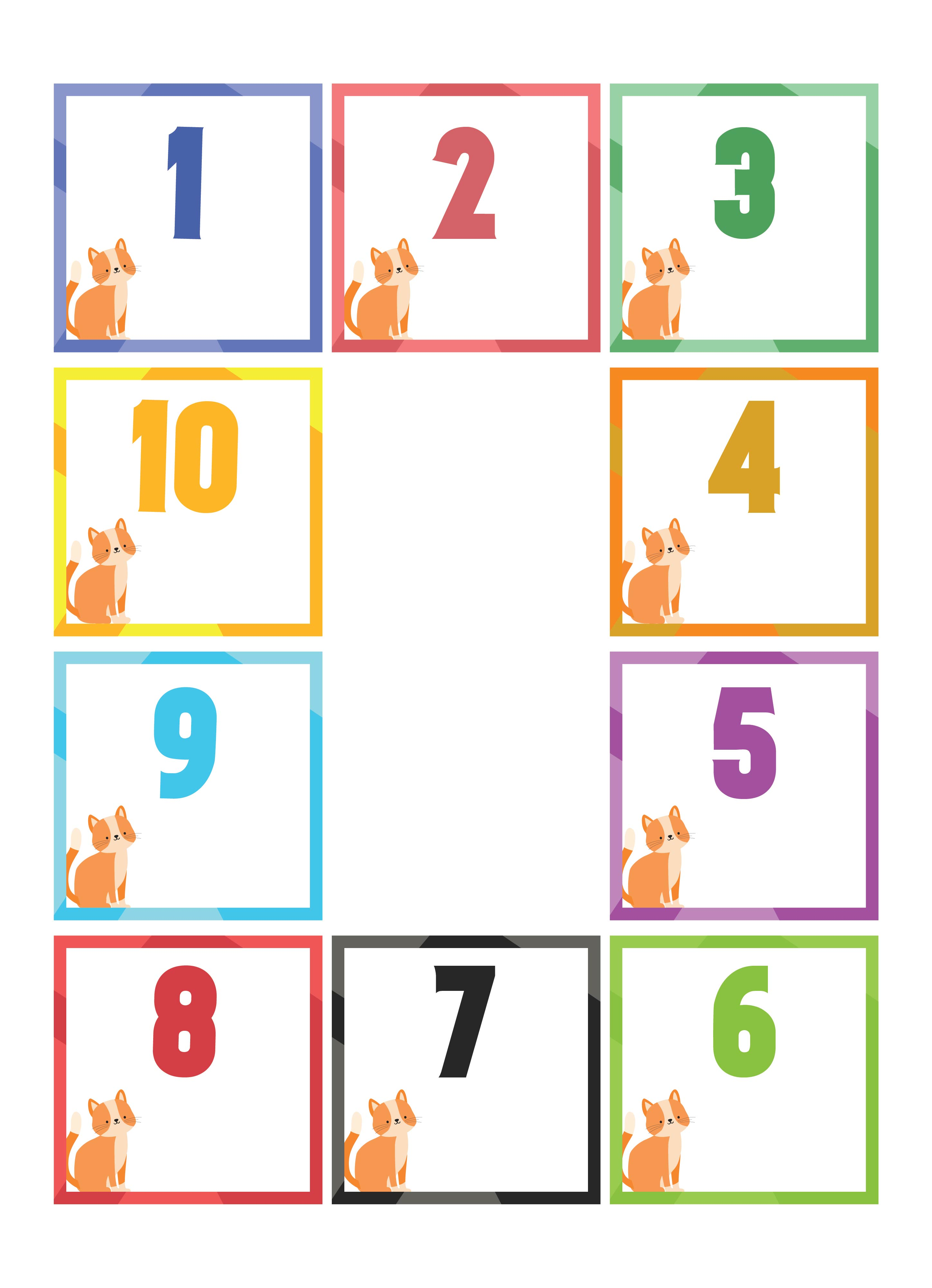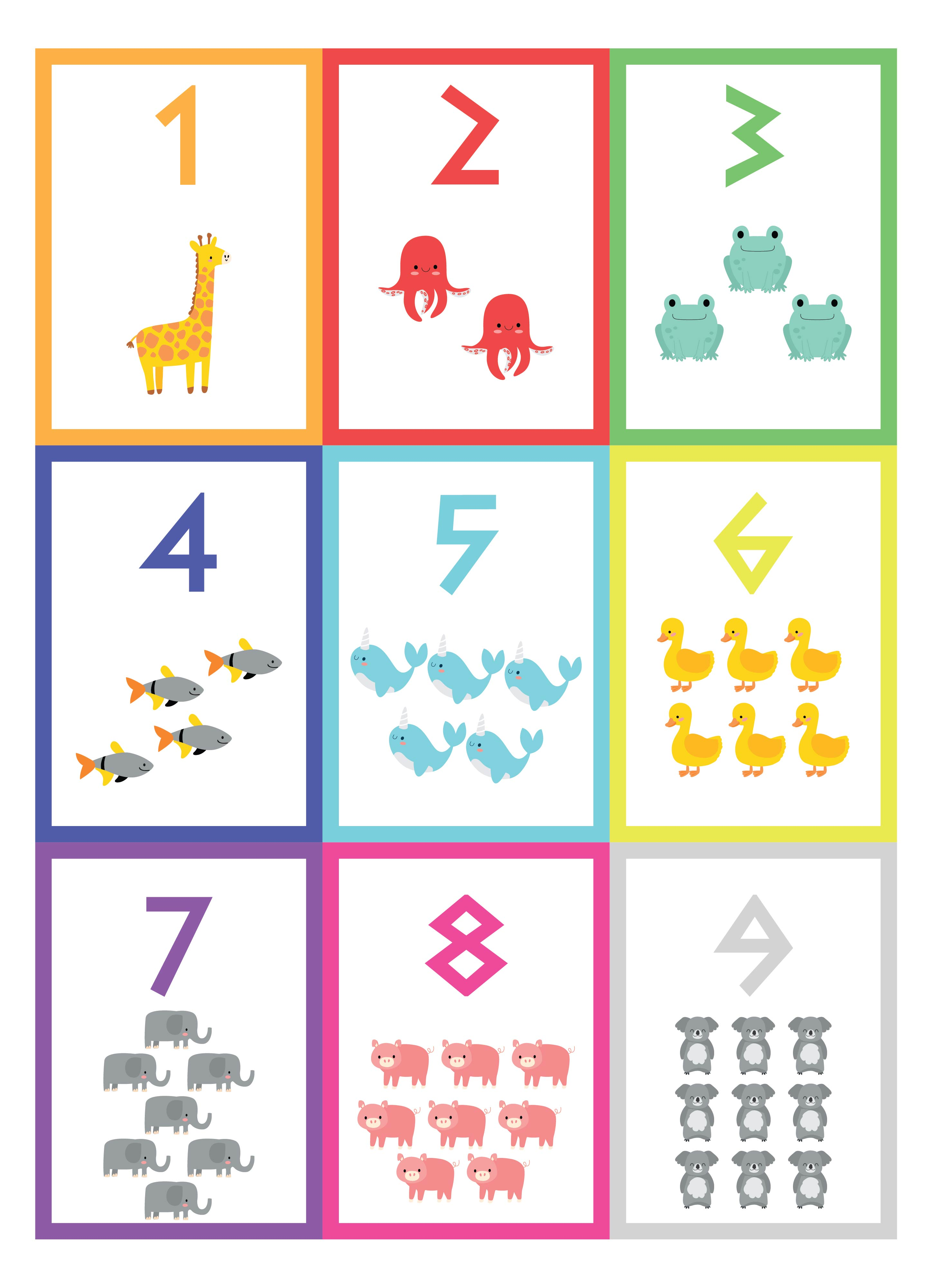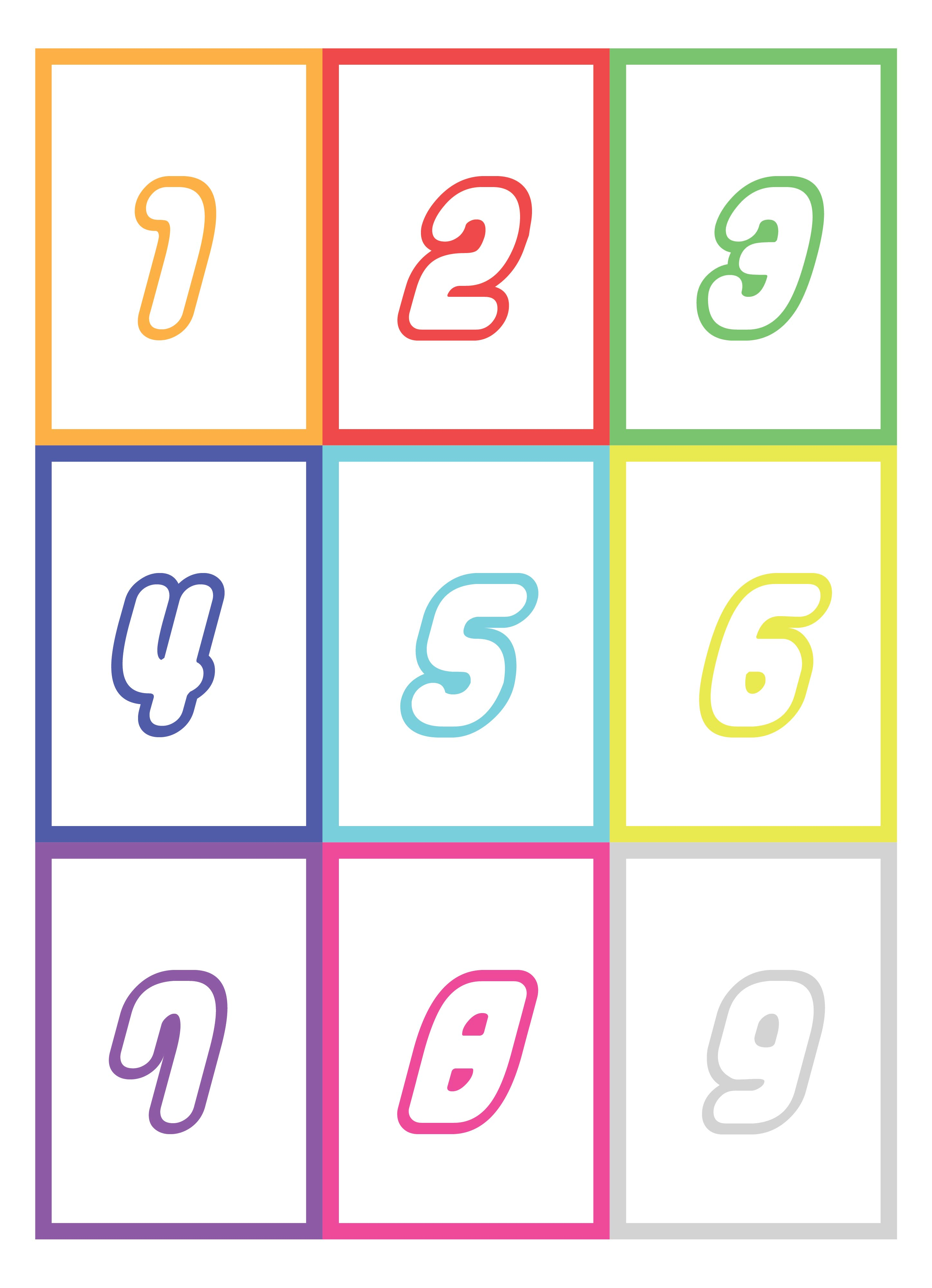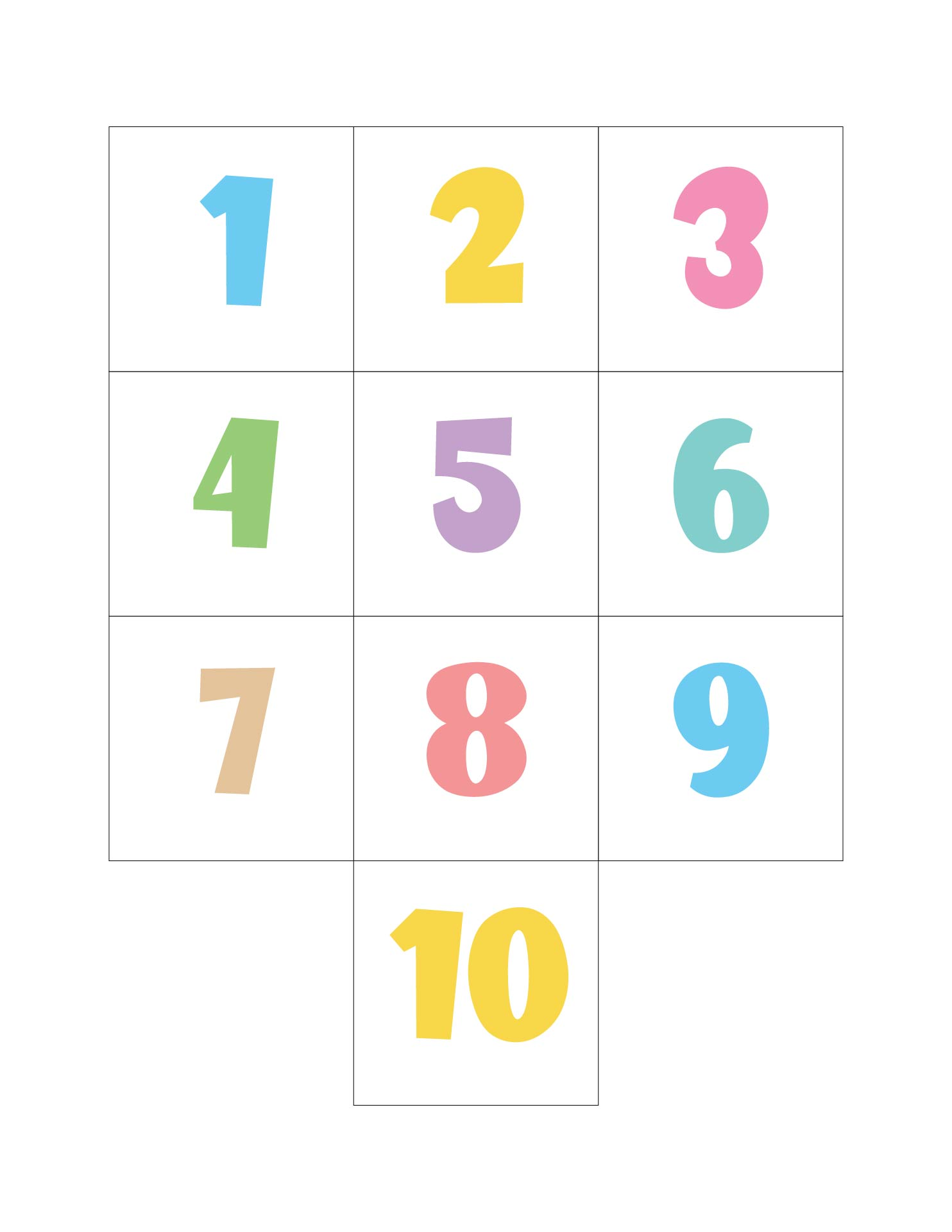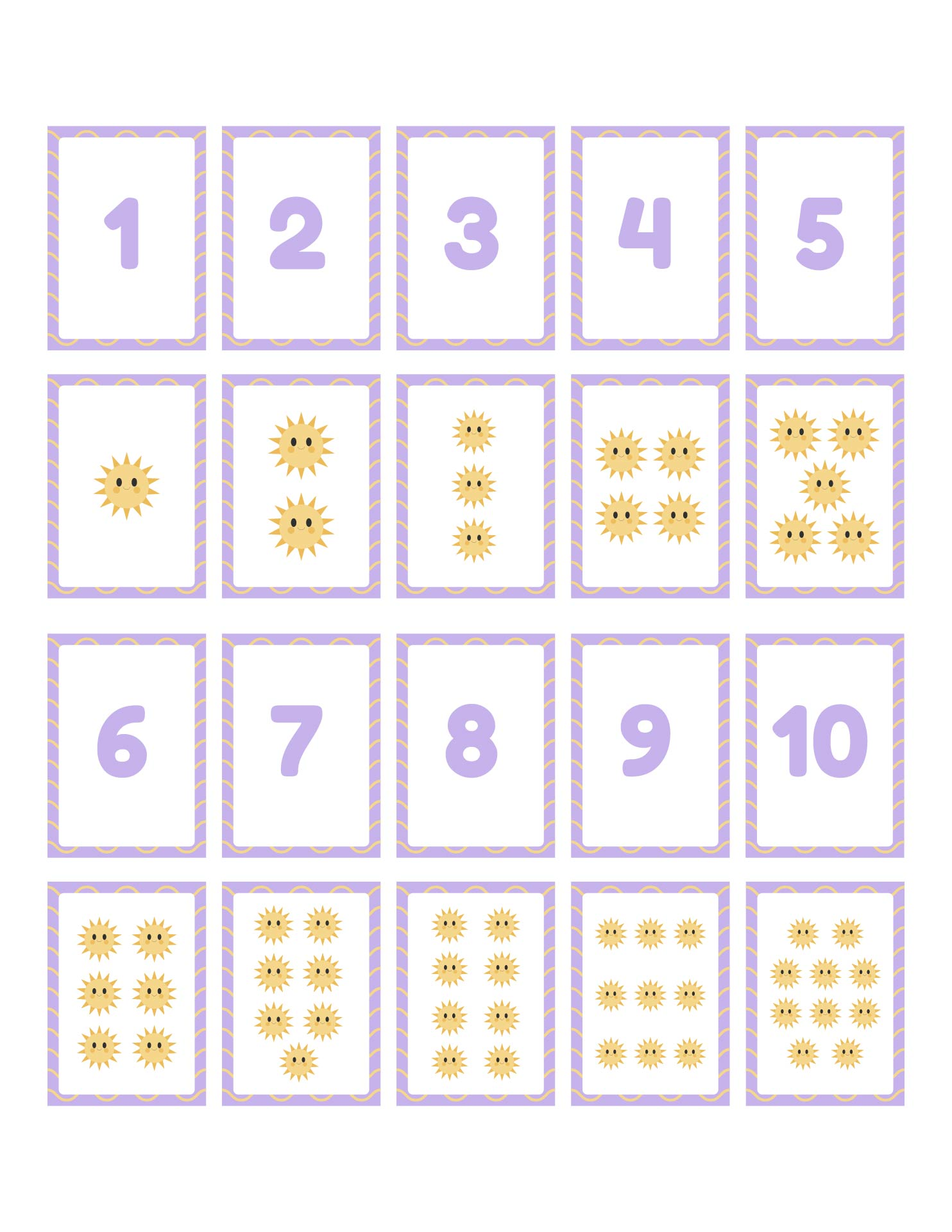### What is the difference between a digit and a number?

As we've discussed before, digit cards 1-10 can make understanding the concept of digits, numbers, and numerals better. However, if we want to teach kids about the difference between the three of them, we need to understand each concept of those. Before we know the concept of the digit, we are required to understand what a number is.

A number refers to a certain measurement that is in a concept of idea inside our mind. Yes, we can write the number 4 to describe a certain amount of the object. However, we can also tap the table as many as four times to indicate that the amount of the object is four. The main importance is number is an idea to determine a certain value. When we want to notice people that there are four birds flying, we write the 4 or four on a piece of paper.

Therefore, the 4 can be defined as a numeral. It is a symbol or name that stands for a number. As we've mentioned above, the digit is one symbol to represent the numerals. For instance, when we want to write the amount of a flying bird, we can write a certain number using a numeral, like 4. However, there is one character there, which is 4. The character or symbol that portrays the numerals is called a digit.